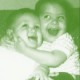MarsEarth

Old world wisdom, new world insight – poems, poetry, philosophy, dreams, commentary, ideasMath geek: Reflections on Statistics

by Lawrence J. J. LeonardMathematics allows the practitioner to practice,
while only a truly devoted student can stud.

~~~

Medicine makes people ill, mathematics make them sad and
theology makes them sinful.”                                                                      ~ Martin Luther (1483-1546)
German professor of theology, composer, priest, former monk

This algebraic formula is also a limerick:Integral t-squared dt
from ONE to the cube root of THREE
times the cosine
of THREE Pi over NINE
equals log of the cube root of ‘E’.
(Yes, it is correct.)

~~~

A mathematician confided
That the Möbius band is one-sided
And you’ll get quite a laugh
If you cut one in half
Because it stays in one piece when divided.

~~~

A graduate student from Trinity
Computed the cube of infinity;
But it gave him the fidgets
To write down all those digits,
So he dropped math and took up divinity.

~~~

A conjecture both deep and profound
Is whether the circle is round;
In a paper by Erdös,
written in Kurdish,
A counterexample is found.
(“Erdös” pronounced ER-dish)

~~~

Death is ninety-nine percent fatal to laboratory rats.

~~~

A statistician is a person who draws a mathematically precise line from an unwarranted assumption to a foregone conclusion.

~~~

Fett’s Law: Never replicate a successful experiment.

~~~

Classification of mathematical problems as ‘linear’ and ‘nonlinear’ is really the same as classifying the Universe as ‘bananas’ and ‘non-bananas.’

~~~

Young man, in mathematics you don’t understand thingsyou just get used to them.”
~John von Neumann (1903-1957)
Hungarian/US mathematician and scientist